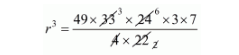# A solid piece of iron of dimensions 49 × 33 × 24 cm`
Question:

A solid piece of iron of dimensions 49 × 33 × 24 cm is moulded into a sphere. The radius of the sphere is

(a) 21 cm

(b) 28 cm

(c) 35 cm

(d) none of these

Solution:

The volume of iron piece = 49 × 33 × 24 cm3

Let, r is the radius sphere.

Clearly,

The volume of sphere = volume of iron piece

$\frac{4}{3} \pi r^{3}=49 \times 33 \times 24$

$\frac{4}{3} \times \frac{22}{7} \times r^{3}=49 \times 33 \times 24$$r^{3}=49 \times 3 \times 3 \times 3 \times 7$

$r=7 \times 3$

$r=21 \mathrm{~cm}$

Hence, the correct answer is choice (a).